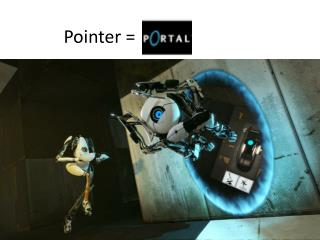DownloadDownload PresentationPointer =

# Pointer =

Télécharger la présentation## Pointer =

- - - - - - - - - - - - - - - - - - - - - - - - - - - E N D - - - - - - - - - - - - - - - - - - - - - - - - - - -
##### Presentation Transcript

1. Pointer =

2. Pointers = Portals? =* Coincidence?

3. Pointer Intro • Data =

4. Variables • Data • Variable “int A” • Int A = Data

5. Pointers = Portals • A pointer is a portal • Whenever you see *, you are using a portal

6. Interactive Section

7. Declare a Pointer • Int * p; • Where does this point? • Nowhere, yet

8. Recap • intfirstvalue=5; • What does this do? • int* mypointer; • What does this do? • Where does it point? • mypointer= &firstvalue; • Is there a *? • Are you going through a portal? • What does this do? • *mypointer = 10; • Is there a *? • Are you going through a portal? • What does this do? • Firstvalue and *mypointer change

9. Recap 2 • char a; • char * b; • char ** c; • a = 'z'; • b = &a; • c = &b; • What does c=? • Did you go through a portal? • What does **c=? • How many portals did you go through?

10. Recap 3 • intfirstvalue=5; • int* mypointer; • mypointer= &firstvalue; • *mypointer++; • Is there a *? • Are you going through a portal? • What does this do? • *mypointer and firstvalue =6; • mypointer++; • Is there a *? • Are you going through a portal? • What does this do?